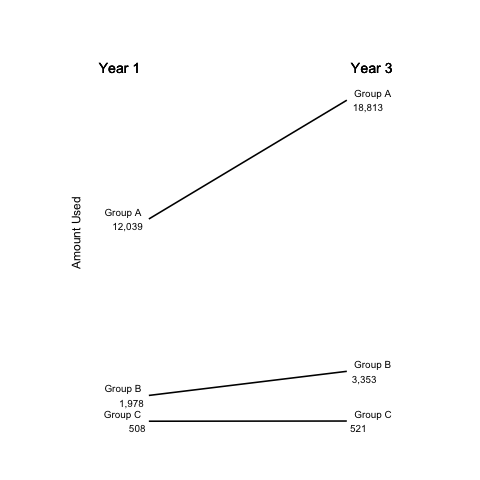27 November 2013

Slopegraphs have seen some recent attention on Edward Tufte's forum and in the data visualization community, especially Charlie Park's excellent treatment of them. In this post, I make a simple slopegraph using less than 20 lines of R and `ggplot2`.

Slopegraphs are very simple—there is no (as ET says) chartjunk.

There are examples of making slopegraphs in ggplot (http://www.jameskeirstead.ca/blog/slopegraphs-in-r/, http://www.r-bloggers.com/slopegraphs-in-r-2/, https://github.com/bobthecat/codebox/blob/master/table.graph.r) all over the web, but what's the web if it doesn't have one more example?

Plus, mine is simple—less than 20 lines of R code if you don't include the initialization of the data.

## Load the libraries

I obviously need the `ggplot2` library, otherwise I would be lying in the title. I also load the `scales` library so I can label the points nicely by putting commas in the numbers.

```library(ggplot2)
library(scales)
```

## Load the data

Likely you'll load the data from a file into a data frame, and it doesn't matter too much what it looks like, as long as each row has the start value, the end value, and the label somewhere in it. Or it could be three different variables in other places.

You'll also need a variable for the x-axis. In my case, this is 24 months (see: `months<-24`)

```months<-24
year1<-c(1338229205,5212325386,31725112511)
year3<-c(1372425378,8836570075,49574919628)
group<-c("Group C", "Group B", "Group A")
a<-data.frame(year1,year3,group)
```

## Set the arrays of labels

This makes the strings we'll use for the labels by combining the `group` name with the value at each end of our slope line.

I also use the `comma_format()()` function from the `scales` library here to make slightly cleaner looking labels.

If you prefered a more Tufte-esque label, you can omit the newline from the `sep=` option and flip the order of the second set of labels.

```l11<-paste(a\$group,comma_format()(round(a\$year1/(3600*24*30.5))),sep="\n")
l13<-paste(a\$group,comma_format()(round(a\$year3/(3600*24*30.5))),sep="\n")
```

## Draw the slopelines

This line is pretty simple but draws the slopelines using the `geom_segment` where the x-range is 0 to `months` and the y-range is from the `a` data frame values.

```p<-ggplot(a) + geom_segment(aes(x=0,xend=months,y=year1,yend=year3),size=.75)
```

## Set the theme to be nothingness

These settings turn off all of the default `ggplot2` decorations (chartjunk?).

```p<-p + theme(panel.background = element_blank())
p<-p + theme(panel.grid=element_blank())
p<-p + theme(axis.ticks=element_blank())
p<-p + theme(axis.text=element_blank())
p<-p + theme(panel.border=element_blank())
```

## Set the axis labels and limits

The x label is empty because we'll use the column headings to denote the time span (or whatever x-range you have), the y label is still useful, though, although the `theme_text(vjust=X)` moves the label a little closer—you'll want to play with this for your own plot until it looks correct to you.

Also, I make the plot area a little bigger by setting `xlim` and `ylim` to be bigger than the data would imply. This is also a bit of art, so you'll want to look at this for your own chart, too.

```p<-p + xlab("") + ylab("Amount Used")
p<-p + theme(axis.title.y=theme_text(vjust=3))
p<-p + xlim((0-12),(months+12))
p<-p + ylim(0,(1.2*(max(a\$year3,a\$year1))))
```

## Label the slopelines

Here we use the labels we created above in the `geom_text()` function, once for each side of the slopeline. The first line below is for the right side (`year3`) of the chart and the second is for the left side (`year1`).

The `rep.int` command that sets `x` just repeats the number—either `0` or `24`—to make the same number of elements in `x` as there are in `y`.

Again, the `hjust` and `size` parameters will need some attention to get your slopegraph to look just right.

```p<-p + geom_text(label=l13, y=a\$year3, x=rep.int(months,length(a)),hjust=-0.2,size=3.5)
p<-p + geom_text(label=l11, y=a\$year1, x=rep.int( 0,length(a)),hjust=1.2,size=3.5)
```

## Label the columns

The columns titles, or labels at the top of each side of the slopegraph, are set here using `geom_text()` and setting `y` to just a bit above the maximum value from the two lists of values (`year1` and `year2`). Don't forget to pay attention to `hjust` and `size` again.

```p<-p + geom_text(label="Year 1", x=0,     y=(1.1*(max(a\$year3,a\$year1))),hjust= 1.2,size=5)
p<-p + geom_text(label="Year 3", x=months,y=(1.1*(max(a\$year3,a\$year1))),hjust=-0.1,size=5)
```

## Finally, some graphics

In the end, this R code produces this figure:## The entire script, suitable for copying-and-pasting is here

```library(ggplot2)
library(scales)
months<-24
year1<-c(1338229205,5212325386,31725112511)
year3<-c(1372425378,8836570075,49574919628)
group<-c("Group C", "Group B", "Group A")
a<-data.frame(year1,year3,group)
l11<-paste(a\$group,comma_format()(round(a\$year1/(3600*24*30.5))),sep="\n")
l13<-paste(a\$group,comma_format()(round(a\$year3/(3600*24*30.5))),sep="\n")
p<-ggplot(a) + geom_segment(aes(x=0,xend=months,y=year1,yend=year3),size=.75)
p<-p + theme(panel.background = element_blank())
p<-p + theme(panel.grid=element_blank())
p<-p + theme(axis.ticks=element_blank())
p<-p + theme(axis.text=element_blank())
p<-p + theme(panel.border=element_blank())
p<-p + xlab("") + ylab("Amount Used")
p<-p + theme(axis.title.y=theme_text(vjust=3))
p<-p + xlim((0-12),(months+12))
p<-p + ylim(0,(1.2*(max(a\$year3,a\$year1))))
p<-p + geom_text(label=l13, y=a\$year3, x=rep.int(months,length(a)),hjust=-0.2,size=3.5)
p<-p + geom_text(label=l11, y=a\$year1, x=rep.int( 0,length(a)),hjust=1.2,size=3.5)
p<-p + geom_text(label="Year 1", x=0,     y=(1.1*(max(a\$year3,a\$year1))),hjust= 1.2,size=5)
p<-p + geom_text(label="Year 3", x=months,y=(1.1*(max(a\$year3,a\$year1))),hjust=-0.1,size=5)
p
```